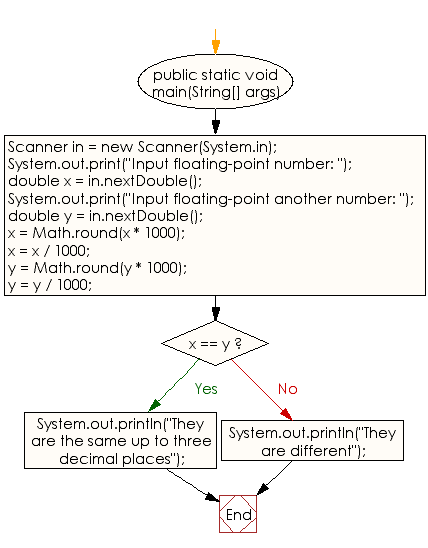﻿ Java - Test 2 floating numbers are same upto 3 decimal places

# Java: Test two floating-point numbers are same upto three decimal places

## Java Conditional Statement: Exercise-6 with Solution

Write a Java program that reads two floating-point numbers and tests whether they are the same up to three decimal places.

Test Data
Input floating-point number: 25.586
Input floating-point another number: 25.589

Sample Solution:

Java Code:

``````import java.util.Scanner;
public class Exercise6 {

public static void main(String[] args)
{
Scanner in = new Scanner(System.in);

System.out.print("Input floating-point number: ");
double x = in.nextDouble();
System.out.print("Input floating-point another number: ");
double y = in.nextDouble();

x = Math.round(x * 1000);
x = x / 1000;

y = Math.round(y * 1000);
y = y / 1000;

if (x == y)
{
System.out.println("They are the same up to three decimal places");
}
else
{
System.out.println("They are different");
}
}
}
```
```

Sample Output:

```Input floating-point number:  25.586
Input floating-point another number:  25.589
They are different
```

Flowchart:Java Code Editor:

What is the difficulty level of this exercise?

Test your Programming skills with w3resource's quiz.

﻿

## Java: Tips of the Day

IsPowerOfTwo

Checks if a value is positive power of two.

To understand how it works let's assume we made a call IsPowerOfTwo(4).

As value is greater than 0, so right side of the && operator will be evaluated.

The result of (~value + 1) is equal to value itself. ~100 + 001 => 011 + 001 => 100. This is equal to value.

The result of (value & value) is value. 100 & 100 => 100.

This will value the expression to true as value is equal to value.

```public static boolean isPowerOfTwo(final int value) {
return value > 0 && ((value & (~value + 1)) == value);
}
```

Ref: https://bit.ly/3sA5d4I

We are closing our Disqus commenting system for some maintenanace issues. You may write to us at reach[at]yahoo[dot]com or visit us at Facebook